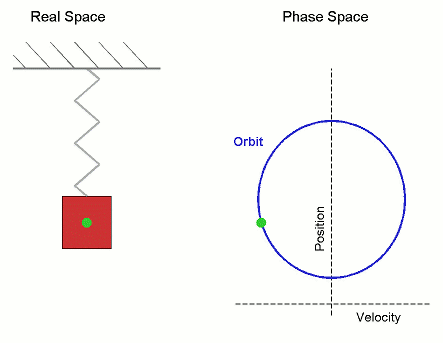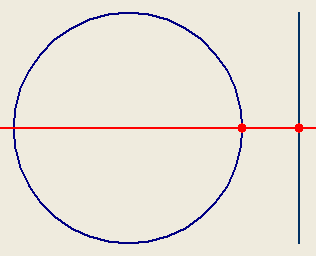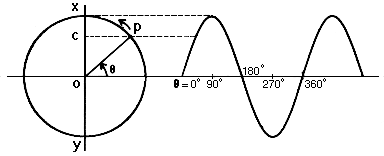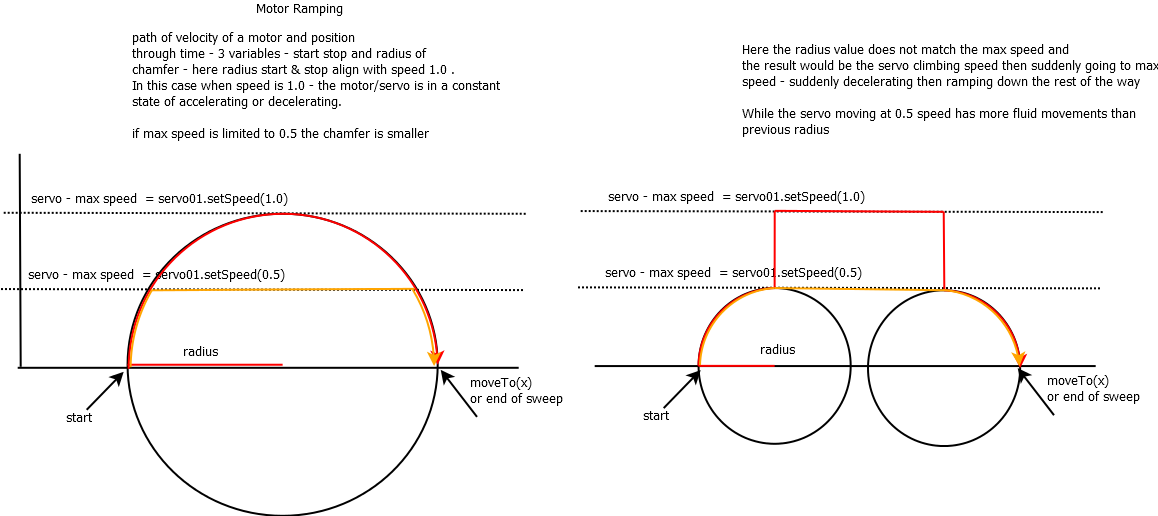# HARMONIC MOTION APPLIED TO SERVO SWEEP

Testing harmonic motion applied to a servo:

The harmonic motion is a type of periodic motion.

For example, the projection of a point moving around a circle on the diameter of the same circle is an example of harmonic motion.The projection of the point moving on the diameter, has not the same speed in every instant

The speed is max near the center and decrease until it arrives to zero at the end, then the speed increase in the opposite verse

The function describing the increase and the inversion of the speed is the SIN FUNCTION

Sin(X), where X is an angle [0;360] , has values between -1 and 1For a servo sweep the amplitude is the difference between servosweepend - servosweepmax, for example 0-180.

So you have an amplitude of 180 degrees, and for example my central point is 90 degrees.

So you can calculate actual degree , servodeg=Amplitude*sin(circle angle in rad) + central position angle

servodeg = 180*sin(circle ang) + 90

You have to iterate that formula for circle angle degrees between 0 and 360, with an increase factor inc (maybe 5 degrees)

References :

www.mauroalfieri.it

Here is a video of how your servo should move:

Here is an application of harmonical motion to a biomechanical hand

[[harmonicalsweep.py]]

## Comment viewing options### Great Science !

We need to make a MRL Servo.sweep(start, stop) method !

with an optional parameter of "type"  ...   Servo.sweep(start, stop, sinusoidal)

Speed control works "pretty well"  - I tested this

servo01.setSpeed(0.5)
servo01.moveTo(0)
sleep(3)
servo01.moveTo(180)
sleep(3)
servo01.moveTo(0)
sleep(3)
servo01.moveTo(180)
sleep(3)
the sinusoidal shoul probably use this same speed concept in MRLComm.ino### Visualized Over Servo Methods !We need another optional variable r = Radius in the servo library !### Radius would be a nice

Radius would be a nice stuff..... Harmonic sweep is becoming really interesting

:D JUST FOR SCIENCE !!! :D